Skip to main content

# Research on task assignment to minimize travel cost for spatio-temporal crowdsourcing

## Abstract

Online task assignment is one of the core research issues of spatio-temporal crowdsourcing technology. The current researches on minimizing travel cost all focus on the scenario of two objectives (task requesters and workers). This paper proposes a two-stage framework (GH) based on Greedy algorithm and Hungarian algorithm for three-objective online task assignment to minimize travel cost. In order to further optimize the efficiency and average travel cost, this paper proposes GH-AT (Adaptive Threshold) algorithm based on GH algorithm, and redesigns the Hungarian algorithm into the sHungarian algorithm. sHungarian algorithm has lower time complexity than Hungarian algorithm. sHungarian algorithm is not only suitable for the problem studied in this paper, but also for all task assignment problems with constraints. Compared with Greedy algorithm, GH-AT algorithm has lower travel cost and higher total utility. In terms of the number of matches, GH-AT is slightly lower than Greedy algorithm. In terms of time cost, GH-AT algorithm is higher than Greedy algorithm, but much lower than GH algorithm.

## Introduction

As crowdsourcing technology becomes more and more widely used in daily life, crowdsourcing applications have become more diverse. Spatio-temporal crowdsourcing is developed from traditional crowdsourcing through combining time and space information .

In Spatio-temporal crowdsourcing, tasks with spatio-temporal data require workers to complete tasks at the specified time and place instead of being completed on the web platform as traditional crowdsourcing .

There are three core study issues for spatio-temporal crowdsourcing: task assignment [3,4,5,6,7], quality control , and privacy protection [9,10,11,12,13,14,15]. Similar to the research of traditional crowdsourcing, incentive mechanism will be one of the important study issues in future [16,17,18,19,20]. In addition, more and more researches also focused on the combination of crowdsourcing and social networks [21, 22] or Big Data . There are also some researches that combine crowdsourcing with blockchain [24, 25].

Earlier studies  focused on the two-objective online task assignment, where tasks are assumed to come dynamically while workers are considered static. A few recent efforts explored the two-objective online task assignment, which has two online objectives. In , authors proposed a two-phase-based framework to maximize the total utility. In , authors summarized all the algorithms, which minimize the total cost. In , authors researched the online task assignment based on task conflict constraints. In , authors applied the multi-armed bandit model to the online task assignment and proposed a heuristic algorithm for spatio-temporal crowdsourcing. In , authors proposed a novel adaptive batch-based solution framework for optimizing the total utility and designed a reinforcement learning based algorithm, which makes near-optimal decisions on batch splitting. The above methods were proposed for two objectives, which cannot adapt the three-objective optimization.

Different from the two-objective online task assignment, some new emerging O2O applications not only consider tasks and workers, but also consider workplaces . As shown by Fig.1, task requester and worker move to workplace to complete task in three-objective online task assignment. For example, Bella wants to haircut in her free time. She had fixed barber and favorite barber shop before. However, it is hardly that Bella, her barber and the barber shop are free at the same time. In fact, there may be some other free barbers and barber shops near her home when she is free. The three-objective online task assignment can match customers, barbers and barber shops in real time.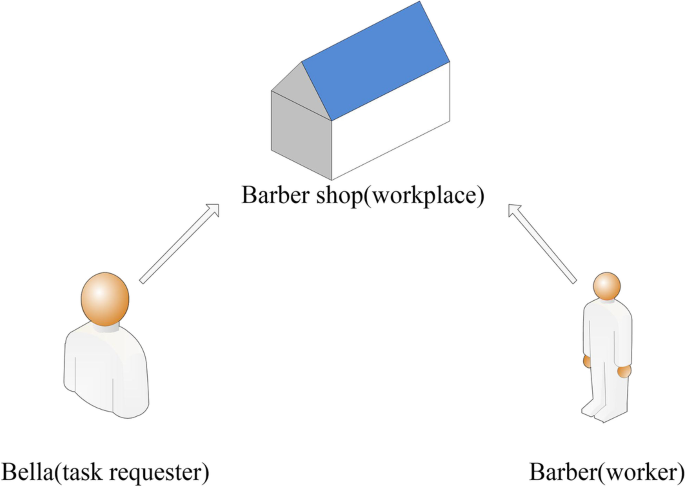Fig. 1

In , authors first studied the three-objective online task assignment and proposed Adaptive RT (Adaptive Random Threshold) algorithm to maximize the total utility. However, Adaptive RT not only has higher time cost, but also ignores the fairness between task requester and workers. Based on the above shortcomings,  quantified fairness as the match quality and proposed QCA (Quality Constraint Algorithm) algorithm to improve the match quality. Although the above researches are based on three objectives, their optimization goal is to maximize the total utility, which is different from the goal of minimizing the travel cost in this paper.

The optimization of the travel cost is extremely meaningful for three-objective online task assignment. Travel costs may be time or money costs in different scenarios. For task requesters, lower time cost means tasks can be executed more timely, and lower money cost means higher cost performance. For workers, lower time cost means more tasks can be completed in the same amount of time, and lower money cost means higher profit. But few researches focus on travel cost for three-objective online task assignment.

In summary, this paper proposes a three-objective online task assignment to minimize the travel cost. To solve the problem, this paper proposes the two-stage GH algorithm based on Greedy algorithm and Hungarian algorithm. In GH algorithm, the assignment process is divided into continuous time windows, and the unmatched objectives are assigned offline at the end of each time window. If the departure time of tasks are earlier than the end time of the current time window, the tasks are assigned based on Greedy algorithm.

Although GH algorithm reduces travel costs well, the efficiency of GH algorithm is not ideal due to the time complexity of Hungarian algorithm . Therefore, this paper proposes GH-AT algorithm based on GH algorithm. In GH-AT algorithm, we improve Hungarian algorithm and add an adaptive threshold mechanism to further improve efficiency and reduce travel cost.

In summary, this paper has the following contributions.

• This paper proposes a three-objective online task assignment method to minimize travel cost.

• This paper proposes a two-stage GH algorithm based on Greedy algorithm and Hungarian algorithm. Then, in view of the shortcomings of GH algorithm, this paper proposes GH-AT algorithm. In GH-AT, we improve Hungarian algorithm and add an adaptive threshold mechanism to further improve the efficiency of GH algorithm and reduce the travel cost.

• The effectiveness and efficiency of the proposed algorithm are verified through comparison experiments on real and synthetic datasets.

## Related work

In this section, the related works on task assignment and Hungarian algorithm are introduced and discussed.

### Task assignment

According to the type of modeling, the task assignment can be divided into the maximum weighted task assignment and the minimum weighted task assignment.

#### Maximum weighted task assignment

Ting et al.  studied the maximum weighted b-matching problem. In the problem, tasks are assumed to come dynamically while workers are static. Ting et al. gave a modification of the randomized algorithm Greedy-RT called Greedy-vRT. However, the above researches were based on a single online objective and failed to apply to many emerging crowdsourcing applications. Tong et al.  studied the more practical task assignment problem, called Global Online Micro-task Allocation (GOMA) in which tasks and workers are assumed to arrive dynamically. Tong et al. proposed a two-phase-based framework with competitive ratio of 1/4 to maximize the total utility and further designed the framework, which runs faster but has lower competitive ratio of 1/8. She et al.  studied the online task assignment based on task conflict constraints and designed two approximation algorithms with provable approximation ratios. In ], authors applied the multi-armed bandit model to the online task assignment and proposed a heuristic algorithm for spatio-temporal crowdsourcing. In , authors proposed a novel adaptive batch-based solution framework for optimizing the total utility and designed a reinforcement learning based algorithm for batch splitting. The above studies were proposed based on two objectives, but failed to adapt the three objectives optimization problem.

With the emergence of more and more crowdsourcing applications, crowdsourcing participants include not only workers and task requesters, but also workplaces. Song et al.  proposed a novel dynamic task assignment problem, called three-objective online task assignment in spatio-temporal crowdsourcing. This problem not only includes the three objectives, but also focuses on dynamic scenarios where tasks, workers and workplaces arrive dynamically. They first proposed Random algorithm to maximize the total utility for the problem. Then, in order to further improve the total utility, they proposed Adaptive RT algorithm. Adaptive RT is a threshold-based randomized algorithm that includes an adaptive optimization technique, which can learn the optimal threshold for the randomized algorithm. However, Adaptive RT algorithm only considers the total utility as a whole, but ignores the fairness for each match. Therefore,  first quantified the fairness as the match quality and proved that the match quality and the total utility are positively correlated. Then,  proposed QCA (Quality Constraint Algorithm) algorithm to improve the match quality.

The problems studied above can be modeled as maximizing weighted bipartite matching problem or maximum weighted tripartite matching problem. They are different from minimum weighted matching problem studied in this paper.

#### Minimum weighted task assignment

In , authors introduced and studied online versions of weighted matching problems, and presented a simple $$2k-1$$ competitive algorithm for online minimum weighted bipartite matching, where 2k is the number of nodes. Tong et al.  presented a comprehensive experimental comparison of the representative algorithms of online minimum matching on real spatio-temporal data. They also found a surprising result that the simple greedy algorithm was significantly more effective than other algorithms. Pan et al.  minimized travel cost while maximizing total utility by agent negotiation strategy. But minimizing travel cost is not the primary goal, result is not ideal. Similarly, Ren et al.  proposed a novel Matrix Completion Technique based Data Collection (MCTDC) scheme to minimize the total cost while guaranteeing the quality of service (QoS) of the tasks. Wang et al.  proposed a novel adaptive batch-based solution framework for optimizing the total utility and designed a reinforcement learning based algorithm for batch splitting. This solution framework can not only optimize the total utility but also minimize the travel cost. This is because Hungarian algorithm in the solution framework can solve both the maximum weighted problem and the minimum weighted problem.

Most of the problems studied above are based on two objectives, but the problem studied in this paper is based on three objectives.

### Hungarian algorithm

The Hungarian method is a combinatorial optimization algorithm that solves the assignment problem in polynomial time. It was developed and published in 1955 by Harold Kuhn, who gave the name Hungarian method because the algorithm was based on the earlier works of two Hungarian mathematicians . The time complexity of the original algorithm was O($${n^3}m$$). Wong  published a version with time complexity O($${n^2}m$$) in 1979, which is also the currently popular version.

Hungarian algorithm can get the lowest cost matching result in the cost matrix. However, the time complexity of Hungarian algorithm is O($${n^2}m$$), which will bring a heavy computational burden when processing large-scale data.

## Problem definition

The following are the relevant definitions and problem descriptions of task assignment to minimize travel cost for three objectives.

• Definition 1:Crowd Task. A crowd task is denoted by $$<l_t,r_t,RP_t,b_t,e_t>$$. $$l_t$$ is the location of the task requester in a 2D space. $$r_t$$ is the radius of the limited circular range of t, whose center is $$l_t$$. $$RP_t$$ is the task reward. $$b_t$$ and $$e_t$$ are the release and leaving time of t, respectively. $$e_t-b_t$$ is the maximum waiting time for crowd tasks. This paper assumes that the maximum waiting time for all tasks is equal.

• Definition 2:Crowd worker. A crowd worker is denoted by $$<l_w,r_w,q_w,b_w>$$. $$l_w$$, $$r_w$$ and $$b_w$$ are the location, the radius and the release time of w, which are similar as those of task. Workers’ skill proficiency is denoted by $$q_w\in (0,1]$$.

• Definition 3:Crowd Workplace. A crowd workplace is denoted by $$<l_p,b_p,c_p>$$. A workplace p has a location $$l_p$$, a release time $$b_p$$ and a capacity $$c_p$$. $$c_p$$ indicates the maximum number of tasks executed in the workplace at the same time.

• Definition 4:Travel Cost. The travel cost of a single match is defined as $$D(t,p,w)=d_{tp}+d_{pw}$$. $$d_{tp}$$ and $$d_{pw}$$ are Euclidean distances between t and w, p and w, respectively.

• Definition 5:Utility. In , the utility of a single match is defined as $$U(t,p,w)=RP_t\times q_w$$. In addition to reward of tasks and skill proficiency of workers, this paper considers that the total utility is also related to the travel cost. The smaller the travel cost, the higher the benefits for worker and task requesters. Therefore, the utility of a single match is defined as $$U(t,p,w)=\frac{RP_t\times q_w}{D(t,p,w)}$$ in this paper.

• Definition 6:Task Assignment to Minimize Travel Costs for Three Objectives. Given a set of tasks T, a set of workers W and a set of workplaces P on crowdsourcing platform, which has no objective initially and allows each objective to arrive dynamically. A match $$<t,p,w>$$ is denoted by m. M is the set of m. The goal of task assignment is to find M among the task set T, the worker set W and the workplace set P to minimize the travel costs MinAveDis(M) shown by 1 and maximize the total utility MaxSumUtility(M) shown by 2.The following constraints should be satisfied. $$\mathbf {Deadline}$$ $$\mathbf {constraint:}$$ tasks should be matched between the release time and the leaving time. $$\mathbf {Invariable}$$ $$\mathbf {constraint:}$$ once the task assignment $$<t,p,w>$$ is given, it cannot be changed. $$\mathbf {Range}$$ $$\mathbf {constraint:}$$ any workplace matched to a task t and a worker w must locate in the restricted radius. $$\mathbf {Capacity}$$ $$\mathbf {constraint:}$$ workplace p can only be matched to $$c_p$$ tasks and $$c_p$$ workers at the same time.

\begin{aligned} MinAveDis(M)= & {} \frac{\sum \nolimits _{t\in T,p\in P,w\in W}D(t,p,w)}{Sum(M)} \end{aligned}
(1)
\begin{aligned} MaxSumUtility(M)= & {} \sum \nolimits _{t\in T,p\in P,w\in W}U(t,p,w) \end{aligned}
(2)

## Methods

Existing researches on task assignment mostly use the real-time assignment strategy. In real-time assignment strategy, for example, when a new task arrives, algorithm immediately looks for an unmatched workplace. The real-time assignment strategy has two shortcomings. First, when each new object arrives, the assignment algorithm will be executed once. This greatly reduces the efficiency. Second, the assignment strategy may miss better matches that are coming soon. In order to avoid the above problems, this paper uses a batch-based assignment strategy. In batch-based assignment strategy, the assignment process is divided into n time windows of equal length. At the end of each time window, task assignment is performed. Based on the above assignment strategy, this paper proposes GH algorithm.

### GH algorithm

In the batch-based assignment strategy, the first problem is how to set the length of time window $$H_n$$. If $$H_n$$ is too short, the batch-based assignment strategy is close to the real-time assignment strategy. If $$H_n$$ is too long, the task will leave the crowdsourcing platform before it is assigned. Therefore, in GH algorithm, the length of time window $$H_n$$ is set to the maximum waiting time of crowd tasks. In this way, the time window can be made long enough, and the objects arrived in the current time window will not leave the crowdsourcing platform before it is assigned.

As shown in Fig. 2, the assignment process is divided into time windows $$H_{n-1}$$, $$H_n$$, $$H_{n+1}$$ and $$H_{n+2}$$ according to time sequence. During the task assignment process, objects arrived in the current time window $$H_n$$ are not assigned immediately, but are assigned at the end of the time window $$H_n$$. At the end of $$H_n$$, the unmatched objects in the crowdsourcing platform include newly arrived objects in $$H_n$$ and objects that arrived in $$H_{n-1}$$ and were not successfully assigned. When assigning unmatched objects, since the information of the unmatched objects is known at this time, the task assignment at the end of the time window $$H_n$$ can be considered as the minimum weighted tripartite matching problem in offline scenarios. Hungarian algorithm can solve the minimum bipartite matching problem in offline scenarios, but there is no applicable algorithm for the minimum weighted tripartite matching problem. To solve this problem, GH algorithm uses a two-stage assignment strategy which decomposes the minimum weighted tripartite matching problem into the minimum weighted bipartite matching problem, as shown in Fig. 3. In the first stage, GH algorithm assigns the unmatched crowd workplaces p and the unmatched crowd task t using Hungarian algorithm. Since the crowd workplaces p has a capacity attribute $$c_p$$, and Hungarian algorithm does not support objects with a capacity attribute, we consider the crowd workplace p with capacity $$c_p$$ as $$c_p$$ same crowd workplaces with capacity 1. $${\widetilde{p}}$$ is the crowd workplaces already assigned to crowd task. In the second stage, GH algorithm assigns $${\widetilde{p}}$$ and the unmatched crowd workers w using Hungarian algorithm.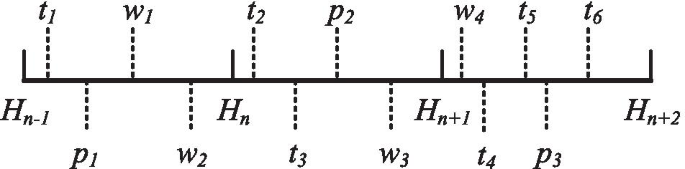Fig. 2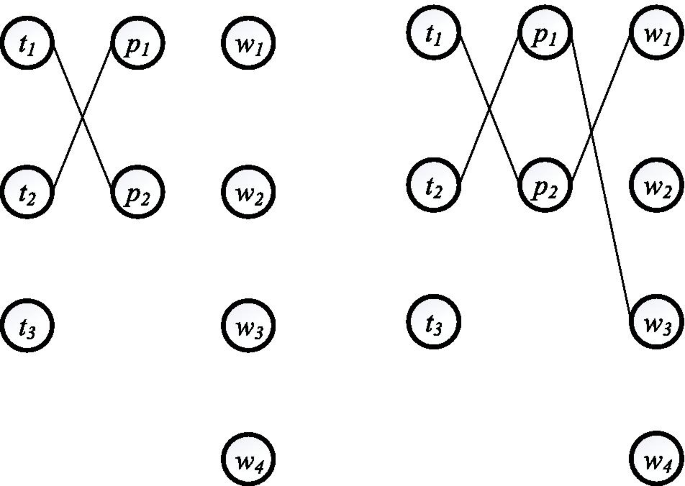Fig. 3

In the first stage, due to the difference of the number of crowd participants and range constraint, the matches obtained by Hungarian algorithm are usually not perfect matching, where all objects are successfully assigned. If the workplace p is not successfully assigned in the current time window, then it will continue to be assigned in the next time window until it is successfully assigned. However, the crowd task t has a leaving time attribute $$e_t$$ and the maximum waiting time is $$e_t-b_t$$, so if the crowd task t is not successfully assigned in the current time window, it will leave the crowdsourcing platform and cannot be assigned anymore. The above problem will cause the total number of matches to be greatly reduced. The reduction of the number of matches will lead to a reduction in the total utility. To solve the above problem, GH algorithm uses Greedy algorithm to assign the crowd task t that arrived in $$H_n$$ and were not successfully assigned at the end of $$H_n$$. The above process is performed within the time window $$H_{n + 1}$$.

In the second stage, there will also be $${\widetilde{p}}$$ and w that have not been successfully assigned. Since p and w do not leave the crowdsourcing platform until they are successfully assigned, they can continue to wait for the next assignment. It should be noted that $${\widetilde{p}}$$ is a optimal match only in the current time window. In the subsequent time window, because the set of unmatched objects has changed, $${\widetilde{p}}$$ is no longer a optimal match. Therefore, before the next assignment, GH algorithm will split $${\widetilde{p}}$$ which is not successfully assigned in the second stage into unmatched p and unmatched t.

The input of Hungarian algorithm is the cost matrix between two objectives shown by 3. It represents Euclidean distance between object i and object j.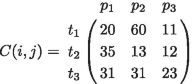(3)

The result obtained by using the above matrix as the input of Hungarian algorithm is $$M={(t_1,p_3),(t_2,p_2),(t_3,p_1)}$$. Assuming the range constraint r is 30, the match $$(t_3,p_1)$$ in the result does not satisfy the range constraint and GH algorithm can only get two valid matches. Hungarian algorithm cannot solve the task assignment problems with constraints that will lead to a reduction in the number of matches. GH algorithm uses the cost setting method shown by 4 and 5 to solve this problem.

\begin{aligned} t_ip_j= & {} \left\{ \begin{aligned} \begin{array}{ll} d_{t_ip_j}, &{}\quad d_{t_ip_j}\le r_{t_i} \\ r_{t_i}\times 10, &{}\quad d_{t_ip_j} > r_{t_i} \end{array} \end{aligned} \right. \end{aligned}
(4)
\begin{aligned} w_ip_j= & {} \left\{ \begin{aligned} \begin{array}{ll} d_{w_ip_j} , &{}\quad d_{w_ip_j}\le r_{w_i} \\ r_{w_i}\times 10, &{}\quad d_{w_ip_j} > r_{w_i} \end{array} \end{aligned} \right. \end{aligned}
(5)

where $$d_{t_ip_j}$$ is Euclidean distance between $$t_i$$ and $$p_j$$. The cost matrix shown by 3 can be transformed into the cost matrix shown by 6 through the above cost setting method.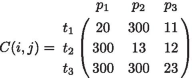(6)

When the cost matrix shown by (6) is used as the input of Hungarian algorithm, the result is $$M={(t_1,p_3),(t_2,p_2),(t_3,p_1)}$$. In M, all matches meet range constraint. Algorithm 1 shows the whole procedure of GH algorithm. The input of the algorithm is the crowd task t, crowd worker w, and crowd workplace p that come dynamically, and the output is a set M of matches. In line 1, GH algorithm divides the assignment process into continuous time windows. In lines 2-7, $$V_c$$ is used to store newly arrived objects in the current time window, $$T_l$$ is used to store tasks that have arrived in the previous time window and have not been successfully assigned. Whenever a new object other than task t arrives, use Greedy algorithm to match it with task t that will leave the crowdsourcing platform. In line 8, GH algorithm deletes the objects that have been successfully assigned and the timeout tasks from the sets. In lines 9-18, If the current time window ends at the current time, GH algorithm uses Hungarian algorithm to assign the objects in the sets $$P_c$$ and $$T_c$$ to get the set $${\widetilde{p}}$$. Then, GH algorithm again uses Hungarian algorithm to assign the objects in the sets $${\widetilde{p}}$$ and $$W_c$$ to get the set $$M_c$$. In line 14, GH algorithm deletes the objects that have been successfully assigned from the sets and moves unmatched t in $$T_c$$ to $$T_l$$.

In summary, GH algorithm divides the assignment process into continuous time windows. At the end of each time window, All unmatched objects are assigned offline. Compared with the real-time assignment strategy, the batch-based assignment strategy not only has higher efficiency, but also can get better assignment results. However, when processing large-scale data, the time cost of GH algorithm is not ideal. Because GH algorithm uses Hungarian algorithm with a time complexity $$O(n^2m)$$ twice in each time window. In order to further improve the efficiency of GH algorithm and reduce the travel cost, this paper designs GH-AT algorithm based on GH algorithm.

### GH-AT algorithm

There two differences between GH-AT and GH algorithm. First, the offline assignment algorithm used is no longer the traditional Hungarian algorithm, but an improved Hungarian algorithm called sHungarian in GH-AT algorithm. Second, GH-AT algorithm adds an adaptive threshold mechanism, which further improves the algorithm efficiency and reduces the travel cost.

#### sHungarian algorithm

In GH algorithm, we implemented Hungarian algorithm under a dense cost matrix and analyzed its operation process. We find that if we set the cost greater than range constraint to 0, the zero elements in the cost matrix are much more than the non-zero elements and the cost matrix becomes a sparse matrix. Table 1 is the density of the $$500\times 500$$ cost matrix under different range constraints in the real data. The definition of matrix density is shown by 7. NZE is the number of non-zero elements in the cost matrix. From Table 1, it can be concluded that the larger the range constraint, the denser the cost matrix. Even at the maximum range constraint, the density is only about 0.21.

Based on the above observations, we consider the cost matrix of the task assignment problem with constraints as a sparse cost matrix and operate the sparse matrix through data structures suitable for sparse matrix such as row compression. This greatly reduces the time complexity of Hungarian algorithm. In addition to the data structures suitable for sparse matrix, the steps of Hungarian algorithm also need to be modified. The modified version proposed in this paper is sHungarian algorithm.

\begin{aligned} density=\frac{NZE}{n\times m} \end{aligned}
(7)

In the data structures suitable for sparse matrix such as row compression, zero elements in cost matrix are not stored when storing sparse matrix. Therefore, in sHungarian algorithm, the cost matrix is shown by 8 and 9.

\begin{aligned} t_ip_j= & {} \left\{ \begin{aligned} \begin{array}{ll} d_{t_ip_j}, &{}\quad d_{t_ip_j}\le r_{t_i} \\ 0, &{}\quad d_{t_ip_j} > r_{t_i} \end{array} \end{aligned} \right. \end{aligned}
(8)
\begin{aligned} w_ip_j= & {} \left\{ \begin{aligned} \begin{array}{ll} d_{w_ip_j} , &{} d_{w_ip_j}\le r_{w_i} \\ 0 , &{}\quad d_{w_ip_j} > r_{w_i} \end{array} \end{aligned} \right. \end{aligned}
(9)

The specific process of sHungarian algorithm is as follows.

• $$\mathbf {Step}$$ $$\mathbf {0:}$$ Create an $$n\times m$$ matrix called the cost matrix in which each element represents the cost of assigning one of n workers to one of m jobs. Rotate the matrix so that there are at least as many columns as rows and let $$k=min(n,m)$$. Variable label is False.

• $$\mathbf {Step}$$ $$\mathbf {1:}$$ For each row of the matrix, find the smallest element and subtract it from every element in its row. Record all addition and subtraction operations and go to Step 2.

• $$\mathbf {Step}$$ $$\mathbf {2:}$$ Find a zero(Z) in the resulting matrix. If there is no starred zero in its row or column, star Z. Repeat for each element in the matrix. Go to Step 3.

• $$\mathbf {Step}$$ $$\mathbf {3:}$$ Cover each column containing a starred zero. If k columns are covered, the starred zeros describe a complete set of unique assignments. In this case, Go to DONE, otherwise, Go to Step 4.

• $$\mathbf {Step}$$ $$\mathbf {4:}$$ Find a uncovered zero and prime it. If there is no starred zero in the row containing this primed zero, Go to Step 5. Otherwise, cover this row and uncover the column containing the starred zero. Continue in this manner until there are no uncovered zeros left. Go to Step 6. If the uncovered positions are all zero elements in the initial cost matrix in Step 0, set the variable label to True and go to Step 6.

• $$\mathbf {Step}$$ $$\mathbf {5:}$$ Construct a series of alternating primed and starred zeros as follows. Let Z0 represent the uncovered primed zero found in Step 4. Let Z1 denote the starred zero in the column of Z0 (if any). Let Z2 denote the primed zero in the row of Z1 (there will always be one). Continue until the series terminates at a primed zero that has no starred zero in its column. Unstar each starred zero of the series, star each primed zero of the series, erase all primes and uncover every line in the matrix. Return to Step 3.

• $$\mathbf {Step}$$ $$\mathbf {6:}$$ If label is False, minval is equal to the minimum value of the uncovered elements in the cost matrix. Add minVal to all covered rows in the matrix, subtract minVal from all uncovered columns, record all addition and subtraction operations and go to Step 4. If label is True, form uncovered elements into a new matrix $$C^{'}(i,j)$$. Set the value of the zero elements in $$C^{'}(i,j)$$ to $$r\times 10$$ and the non-zero elements to the initial value before the addition and subtraction operation. Perform all recorded addition and subtraction operations on $$C^{'}(i,j)$$. Then, minval is equal to the minimum value in the matrix $$C^{'}(i,j)$$. If the position of minval is zero elements in the original matrix $$C^{'}(i,j)$$, set the value of the corresponding position in $$C^{'}(i,j)$$ to minval. Add minval to all covered rows, subtract minval from all uncovered columns in $$C^{'}(i,j)$$, record all addition and subtraction operations and go to Step 4.

In summary, sHungarian algorithm converts the cost matrix of task assignment problem with constraints into a sparse matrix, and uses a data structure suitable for the sparse matrix, which reduce the time complexity of Hungarian algorithm from $$O(n^2m)$$ to O(nk). k is the number of non-zero elements in the sparse matrix. sHungarian algorithm is not only suitable for the problem studied in this paper, but also for all task assignment problems with constraints.

#### Adaptive threshold mechanism

sHungarian algorithm has the following two characteristics. First, the efficiency of sHungarian algorithm is related to the density of the cost matrix. Second, although sHungarian algorithm can reduce the average travel cost, there are still some matches with higher travel costs.

Based on the above two characteristics, we can further improve the efficiency of sHungarian algorithm and reduce the average travel cost. GH-AT algorithm adds threshold $$\theta \times r$$ to the cost matrix. If $$d_{t_ip_j} > \theta \times r$$, set $$t_ip_j$$ in the cost matrix to 0. Adding the threshold can not only further reduce the density of the matrix, improve the efficiency of the algorithm, but also eliminate the matches with higher travel costs. The objects in eliminated matches may be better assigned in subsequent assignment.

The value of the threshold directly affects the performance of the algorithm. Too small a threshold will cause too many matches to be eliminated. Too large a threshold will cause the effect of setting the threshold to be not obvious. GH-AT algorithm adopts an adaptive threshold mechanism, which adaptively selects a threshold in the threshold pool according to the cost matrix.

First, set the value range of $$\theta$$ to the closed interval shown by

\begin{aligned} \theta \in \left[ \frac{\lceil \frac{10\times AveDis}{r} \rceil }{10},0.9\right] \end{aligned}
(10)

AveDis is the average travel cost of the result set obtained by Greedy algorithm. For example, AveDis is 6.5 and range constraint r is 10, then $$\theta \in \{0.7,0.8,0.9\}$$. If the value of $$\theta$$ is less than $$\frac{\lceil \frac{10\times AveDis}{r} \rceil }{10}$$, the matches eliminated by $$\theta$$ is most likely to fail in the subsequent matching process, which will cause the total number of matches to be greatly reduced. If the value of $$\theta$$ is 1, the threshold mechanism will have no effect. Before using sHungarian for assignment, GH-AT algorithm first calculates the weight $$w_l$$ of each threshold $${\theta }_l$$ in the threshold pool according to the cost matrix. The weight is calculated by

\begin{aligned} w_l=1-\frac{\left| \{t_i|\varphi _i> \theta _l\times r\}\right| +\left| \{p_j|\varphi _j> \theta _l\times r\}\right| }{\left| \{d_{t_ip_j}|d_{t_ip_j} > {\theta }_l\times r\}\right| } \end{aligned}
(11)

where $$\varphi _i$$ represents the average travel cost between the ith object and all objects in the other category. For example, for task $$t_i$$, $$\varphi _i$$ represents the average travel cost between task $$t_i$$ and all workplaces in the cost matrix. $$\varphi _i$$ is calculated by

\begin{aligned} \varphi _i=\frac{\sum _{j=1}^{\left| P_c\right| }d_{t_ip_j}}{\left| P_c\right| } \end{aligned}
(12)

In (11), the denominator represents the number of travel costs in the cost matrix that is greater than the threshold. The smaller the denominator, the more sparse the cost matrix is, and the faster the algorithm is. The numerator represents the number of objects in the matches that sHungarian algorithm may eliminate when the current threshold is selected. The smaller the numerator, the fewer objects eliminated, and the greater the total number of matches. Therefore, when GH-AT algorithm selects a threshold from the threshold pool, it also selects the threshold with the largest weight.

In summary, GH-AT algorithm uses an improved version of Hungarian algorithm. Compared with GH algorithm, it greatly improves the efficiency of the algorithm. Then, GH-AT adds an adaptive threshold mechanism, which further improves the efficiency of the algorithm and reduces the travel costs.

## Results and discussion

The experiments in this section are divided into two parts. In the first part, the performance of sHungarian algorithm is verified on the real dataset named gMission , and the comparison algorithm is Hungarian algorithm. In the second part, a large number of experiments are performed on the gMission dataset and synthetic dataset to verify the performance of GH-AT algorithm. The comparison algorithms are GH algorithm and Greedy algorithm. The statistics and configuration of synthetic data are illustrated in Table 2, where default setting is marked in bold font.

The distribution of the synthetic dataset is extended on the basis of the gMission dataset. For example, the maximum wait time of tasks on gMission dataset is 30. The maximum wait time of tasks on synthetic dataset is set to 20,25,30,35 and 40. The purpose is to ensure that the distribution of dataset is close to the real situation and study the performance of GH-AT algorithm on various distributed datasets. For the parameters that have no effect on travel costs, we set them as fixed values. Tasks’ rewards and workers’ skill proficiency follow a normal distribution. Locations and release time of objects follow a uniform distribution.

### sHungarian algorithm

This comparison experiments are conducted to verify the efficiency of sHungarian algorithm in the task assignment problem with constraints on gMission data. gMission data is divided into 5 datasets according to the range constraints, which are the datasets with range constraints of 10, 15, 20, 25, and 30. The experimental results are shown in Fig. 4.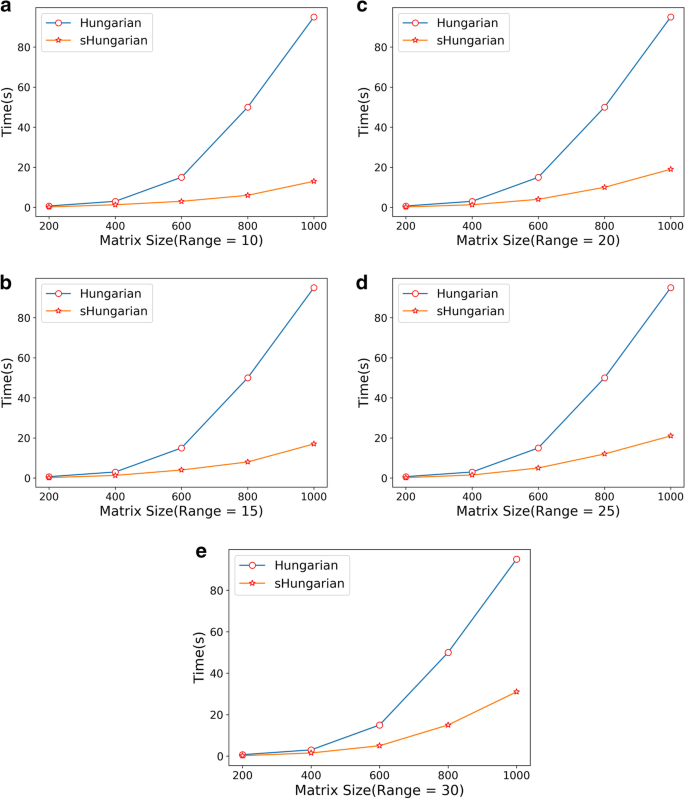Fig. 4

The x-coordinate represents the size of square matrix, and the y-coordinate represents the running time of the algorithms. It can be seen from Fig. 4 that when the square matrix is larger, the running time gap between the two algorithms also increases. In this experiments, the minimum running time of sHungarian algorithm is only 9.1% of Hungarian algorithm. Therefore, in large-scale data processing, sHungarian algorithm is far superior to Hungarian algorithm. As the range constraints and square matrix size increase, the running time of sHungarian algorithm increases faster and faster. This is because the time complexity of the sHungarian algorithm is O(nk), and k is the number of non-zero elements in the sparse matrix. As the range constraints increases, the more non-zero elements in the matrix, the higher the time complexity.

### GH-AT algorithm

We conduct the comparison experiments for the average travel costs, the algorithm efficiency, the number of matches, and the total utility of GH algorithm, GH-AT algorithm, and Greedy algorithm on gMission dataset and synthetic dataset.

#### Effect of cardinality

In this experiment, the effect of cardinality is studied. The experimental results of varying $$\left| T\right|$$ are shown in Fig. 5. The x-coordinate represents $$\left| T\right|$$ and $$\left| T\right| =\left| W\right| =10\times \left| P\right|$$. The y-coordinate represents the average travel cost, total utility, number of matches and time cost of algorithms, respectively.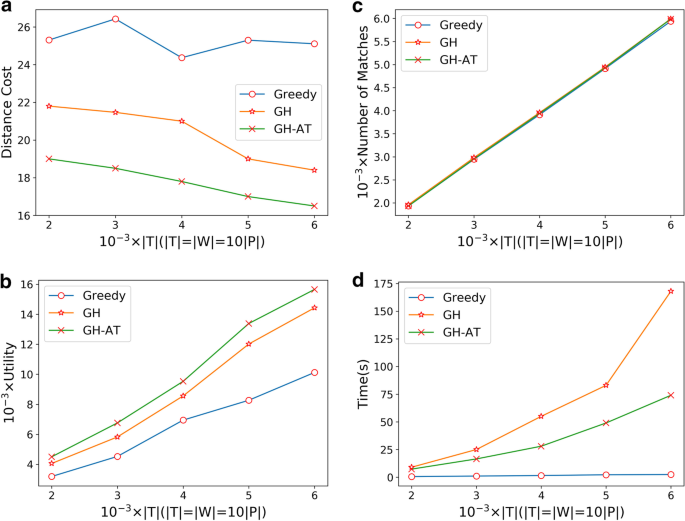Fig. 5

The purposes of the experiments are to compare the performance of the average travel cost, total utility, number of matches and time cost of the three algorithms under different data sizes.

In terms of average travel cost, GH-AT algorithm is 13% lower than GH algorithm and 30% lower than Greedy algorithm on average. It can be seen from Fig. 5 that with the increase of the cardinality, the average travel cost of GH algorithm and GH-AT algorithm is decreasing. This is because the increase of the cardinality leads to an increase in the amount of objects in each time window, and the better the effect of Hungarian algorithm and sHungarian algorithm.

In terms of total utility, GH-AT algorithm is 15% higher than GH algorithm and 53% higher than Greedy algorithm. This is because lower travel costs mean higher total utility. It can be seen from Fig. 5 that as the cardinality increases, the total utility of the three algorithms increases. This is because the number of matches increases as the cardinality increases.

In terms of number of matches, it can be seen from Fig. 5 that the number of matches of the three algorithms seems to be equal. In fact, GH algorithm is slightly higher than Greedy algorithm and GH-AT algorithm. This is because GH algorithm and GH-AT algorithm use a batch-based assignment strategy, which can avoid missing some matches. Because of the threshold mechanism, GH-AT algorithm has a lower number of matches than the Greedy algorithm.

In terms of time cost, GH-AT algorithm and GH algorithm are higher than Greedy algorithm. Compared with GH algorithm, the time cost of GH-AT algorithm is reduced by 41% on average.

#### Effect of range constraint

In this experiment, the effect of range constraint is studied. The experimental results of varying range are shown in Fig. 6. The x-coordinate represents the range constraint of tasks and workers. The y-coordinate represents the average travel cost, total utility, number of matches and time cost of algorithms, respectively.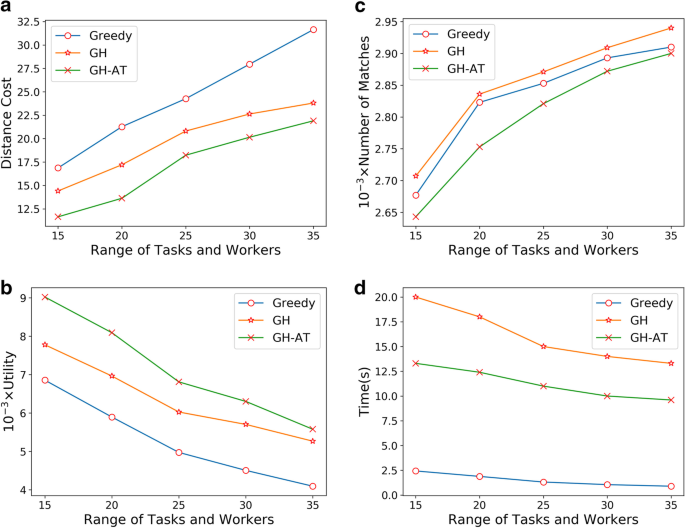Fig. 6

The purposes of the experiments are to compare the performance of the average travel cost, total utility, number of matches and time cost of the three algorithms under different range constraints.

In terms of average travel cost, GH-AT algorithm is 14.8% lower than GH algorithm and 33% lower than Greedy algorithm. With the increase of the range constraint, the advantages of GH-AT algorithm become more and more obvious compared with Greedy algorithm.

In terms of total utility, GH-AT algorithm is 13% higher than GH algorithm and 31% higher than Greedy algorithm. As the range increases, the total utility of the three algorithms decreases overall. This is because as the range increases, the average travel cost of the three algorithms increases.

In terms of number of matches, as the range increases, the number of matches for all three algorithms is increasing. This is because the increase in range constraints leads to an increase in the number of matches that meet the constraints.

In terms of time cost, GH-AT algorithm and GH algorithm are higher than Greedy algorithm. Compared with GH algorithm, the time cost of GH-AT algorithm is reduced by 31% on average. With the increase of the range, the time cost of the three algorithms is gradually decreasing. This is because the larger the range, the more matches that meet the range constraints, and the faster the speed of assignment. The smaller the range, the easier it is for objects to pile up, and each object may be assigned multiple times.

#### Effect of waiting time

In this experiment, the effect of waiting time of tasks is studied. The experimental results of varying waiting time are shown in Fig. 7. The x-coordinate represents the waiting time of tasks. The y-coordinate represents the average travel cost, total utility, number of matches and time cost of algorithms, respectively.Fig. 7

The purpose of the experiments are to compare the performance of the average travel cost, total utility, number of matches and time cost of the three algorithms under different waiting time.

In terms of average travel cost, GH-AT algorithm is 28% lower than GH algorithm and 30% lower than Greedy algorithm. It can be seen from Fig. 7 that as the waiting time increases, the average travel cost of GH-AT algorithm and GH algorithm is decreasing. This is because the length of the time window increases, resulting in an increase in the number of objects arriving within each time window, and the better the effect of Hungarian algorithm and sHungarian algorithm.

In terms of total utility, GH-AT algorithm is 13% higher than GH algorithm and 35% higher than Greedy algorithm. It can be seen from Fig. 7 that as the waiting time increases, the total utility of both GH-AT algorithm and GH algorithm increases. This is because as the waiting time increases, the average travel cost of GH-AT algorithm and GH algorithm are decreasing.

In terms of number of matches, GH-AT algorithm is lower than GH algorithm and Greedy algorithm due to threshold mechanism. With the increase of waiting time, the number of matches of all three algorithms is increasing. This is because the increase of waiting time leads to more time for crowd task t to wait for the assignment to satisfy the constraint, which reduces the number of tasks leaving the crowdsourcing platform.

In terms of time cost, GH-AT algorithm and GH algorithm are higher than Greedy algorithm. compared with GH algorithm, GH-AT is 30% lower than GH algorithm. As the waiting time increases, the time cost of GH algorithm and GH-AT algorithm also increases. This is because the increase of the time window causes the cost matrix to become larger.

#### Experiment on gMission dataset

In this experiment, the result on real dataset is studied. The gMission dataset can be divided into five parts according to the range constraint of tasks and workers. The experimental results of the five parts are shown in Fig. 8. The purpose of the experiments are to compare the performance of the average travel cost, total utility, number of matches and time cost of the three algorithms on the real dataset.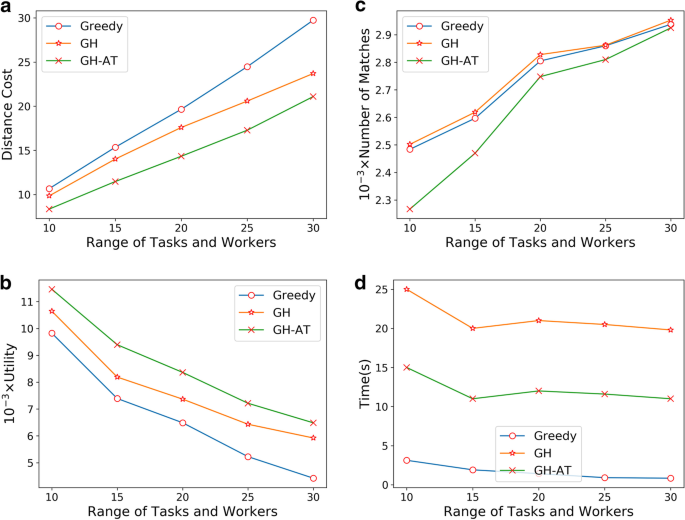Fig. 8

In terms of average travel cost, GH-AT is 7% lower than GH algorithm and 24.8% lower than Greedy algorithm. As the range constraint increases, the average travel cost of all three algorithms increases.

In terms of total utility, GH-AT algorithm is 13% higher than GH algorithm and 31.1% higher than Greedy algorithm. As the range constraint increases, the total utility of the three algorithms decreases, because the travel cost of the three algorithms increases as the range constraint increases.

In terms of number of matches, GH-AT algorithm is lower than GH algorithm and Greedy algorithm due to the threshold mechanism. As the range increases, the number of matches for all three algorithms is increasing. This is because the increase in range constraints leads to more matches meet the constraints.

In terms of time cost, GH-AT algorithm and GH algorithm is higher than Greedy algorithm. Compared with GH algorithm, the time cost of GH-AT algorithm is reduced by 41% on average. With the increase of the range, the time cost of the three algorithms is gradually decreasing. This is because the larger the range, the more matches that meet the range constraints, and the faster the speed of assignment. The smaller the range, the easier it is for objects to pile up, and each object may be assigned multiple times.

### Experiment summary

The experiment of this paper is divided into two parts. First, the performance of the sHungarian algorithm is verified on a real dataset. The experimental results show that compared with Hungarian algorithm, the running time of the sHugarian algorithm can be reduced by more than 90%.

Then, the performance of GH-AT algorithm is verified on real and synthetic dataset. It is found that GH-AT algorithm is always superior to GH algorithm and Greedy algorithm in terms of average travel cost and total utility. In terms of number of matches, GH and Greedy algorithm are slightly higher than GH-AT algorithm, which is due to the adaptive threshold mechanism of the GH-AT algorithm. In terms of the time cost of the algorithm, because of the improvement of Hungarian algorithm and the adaptive threshold mechanism, the average time cost of GH-AT algorithm is far lower than GH algorithm, and GH-AT algorithm and GH algorithm are higher than Greedy algorithm, but the time cost of the GH-AT algorithm processing a single time window can fully meet the real-time requirements of real applications.

## Conclusion

In this paper, a three-objective online task assignment to minimize the travel cost is proposed. In order to solve the problem, this paper proposes a two-stage solution framework based on Greedy algorithm and Hungarian algorithm, which is the GH algorithm. In order to further improve the performance and efficiency of GH algorithm, this paper designs GH-AT algorithm based on GH algorithm. In GH-AT algorithm, the offline assignment algorithm used is no longer Hungarian algorithm, but an improved version based on the Hungarian algorithm. In addition, GH-AT algorithm also adds an adaptive threshold mechanism, which further reduces the time cost of the algorithm and reduces the average travel cost. Finally, through experiments on real and synthetic dataset, this paper proves that the proposed algorithm is not only better than other algorithms, but also has the time overhead that can meet the real-time requirements.

In future work, we will further consider the impact of certain human behaviors on the three-objective task assignment, such as free-riding and false-reporting problems due to selfish behaviors.

## Availability of data and materials

The datasets used and analysed during the current study are available from the corresponding author on reasonable request.

## Abbreviations

GH:

Greedy Hungrian algorithm

GH-AT:

Greedy Hungrian-Adaptive Threshold algorithm

## References

1. 1.

M. Musthag, D. Ganesan, Labor dynamics in a mobile micro-task market. In: Proceedings of the SIGCHI Conference on Human Factors in Computing Systems. CHI’13, pp. 641–650. Association for Computing Machinery, New York, NY, USA (2013). https://doi.org/10.1145/2470654.2470745

2. 2.

Y. Tong, Y. Yuan, Y. Cheng, L. Chen, G. Wang, Survey on spatiotemporal crowdsourced data management techniques. J. Softw. 28(1), 35–58 (2017)

3. 3.

P. Cheng, X. Lian, L. Chen, J. Han, J. Zhao, Task assignment on multi-skill oriented spatial crowdsourcing. IEEE Trans. Knowl. Data Eng. 28(8), 2201–2215 (2016)

4. 4.

H. To, C. Shahabi, L. Kazemi, A server-assigned spatial crowdsourcing framework. ACM Trans. Spat. Algorithms Syst. (2015). https://doi.org/10.1145/2729713

5. 5.

P. Cheng, X. Lian, Z. Chen, R. Fu, L. Chen, J. Han, J. Zhao, Reliable diversity-based spatial crowdsourcing by moving workers. Proc. VLDB Endowment. 8(10), 1022–1033 (2015). https://doi.org/10.14778/2794367.2794372

6. 6.

Y. Wang, Z. Cai, Z. Zhan, B. Zhao, X. Tong, L. Qi, Walrasian equilibrium-based multiobjective optimization for task allocation in mobile crowdsourcing. IEEE Trans. Comput. Soc. Syst. 7(4), 1033–1046 (2020). https://doi.org/10.1109/TCSS.2020.2995760

7. 7.

L. Wang, X. Zhang, T. Wang, S. Wan, G. Srivastava, S. Pang, L. Qi, Diversified and scalable service recommendation with accuracy guarantee. IEEE Trans. Comput. Soc. Syst. (2020). https://doi.org/10.1109/TCSS.2020.3007812

8. 8.

F. Daniel, P. Kucherbaev, C. Cappiello, B. Benatallah, M. Allahbakhsh, Quality control in crowdsourcing: a survey of quality attributes, assessment techniques, and assurance actions. ACM Comput. Surv. (2018). https://doi.org/10.1145/3148148

9. 9.

Y. Wang, Z. Cai, G. Yin, Y. Gao, X. Tong, G. Wu, An incentive mechanism with privacy protection in mobile crowdsourcing systems. Comput. Netw. 102, 157–171 (2016). https://doi.org/10.1016/j.comnet.2016.03.016

10. 10.

Y. Wang, Z. Cai, X. Tong, Y. Gao, G. Yin, Truthful incentive mechanism with location privacy-preserving for mobile crowdsourcing systems. Comput. Netw. 135, 32–43 (2018). https://doi.org/10.1016/j.comnet.2018.02.008

11. 11.

Z. Cai, X. Zheng, A private and efficient mechanism for data uploading in smart cyber-physical systems. IEEE Trans. Netw. Sci. Eng. 7(2), 766–775 (2020)

12. 12.

T. Liu, Y. Wang, Y. Li, X. Tong, L. Qi, N. Jiang, Privacy protection based on stream cipher for spatio-temporal data in iot. IEEE Internet Things J. (2020). https://doi.org/10.1109/JIOT.2020.2990428

13. 13.

L. Qi, C. Hu, X. Zhang, M. Khosravi, S. Sharma, S. Pang, T. Wang, Privacy-aware data fusion and prediction with spatial-temporal context for smart city industrial environment. IEEE Trans. Ind. Inf. (2020). https://doi.org/10.1109/TII.2020.3012157

14. 14.

X. Chi, C. Yan, H. Wang, W. Rafique, L. Qi, Amplified lsh-based recommender systems with privacy protection. Concurr. Comput. Pract. Exp. (2020)

15. 15.

W. Zhong, X. Yin, X. Zhang, S. Li, W. Dou, R. Wang, L. Qi, Multi-dimensional quality-driven service recommendation with privacy-preservation in mobile edge environment. Comput. Commun. 157, 116–123 (2020). https://doi.org/10.1016/j.comcom.2020.04.018

16. 16.

Y. Wang, Y. Li, Z. Chi, X. Tong, The truthful evolution and incentive for large-scale mobile crowd sensing networks. IEEE Access 6, 51187–51199 (2018)

17. 17.

Y. Hu, Y. Wang, Y. Li, X. Tong, An incentive mechanism in mobile crowdsourcing based on multi-attribute reverse auctions. Sensors 18, 3453 (2018). https://doi.org/10.3390/s18103453

18. 18.

J. Li, Z. Cai, J. Wang, M. Han, Y. Li, Truthful incentive mechanisms for geographical position conflicting mobile crowdsensing systems. IEEE Trans. Comput. Soc. Syst. 5(2), 324–334 (2018). https://doi.org/10.1109/TCSS.2018.2797225

19. 19.

Y. Wang, Y. Gao, Y. Li, X. Tong, A worker-selection incentive mechanism for optimizing platform-centric mobile crowdsourcing systems. Comput. Netw. 171, 107144 (2020). https://doi.org/10.1016/j.comnet.2020.107144

20. 20.

J. Xu, S. Wang, N. Zhang, F. Yang, X. Shen, Reward or penalty: Aligning incentives of stakeholders in crowdsourcing. IEEE Trans. Mob. Comput. 18(4), 974–985 (2019). https://doi.org/10.1109/TMC.2018.2847350

21. 21.

J.L.Z. Cai, M. Yan, Y. Li, Using crowdsourced data in location-based social networks to explore influence maximization. In: IEEE INFOCOM 2016 - The 35th Annual IEEE International Conference on Computer Communications, pp. 1–9 (2016). https://doi.org/10.1109/INFOCOM.2016.7524471

22. 22.

J. Li, T. Cai, K. Deng, X. Wang, T. Sellis, F. Xia, Community-diversified influence maximization in social networks. Inf. Syst. 92, 101522 (2020). https://doi.org/10.1016/j.is.2020.101522

23. 23.

L. Qi, W. Dou, C. Hu, Y. Zhou, J. Yu, A context-aware service evaluation approach over big data for cloud applications. IEEE Trans. Cloud Comput. 8(2), 338–348 (2020). https://doi.org/10.1109/TCC.2015.2511764

24. 24.

Y. Xu, J. Ren, Y. Zhang, C. Zhang, B. Shen, Y. Zhang, Blockchain empowered arbitrable data auditing scheme for network storage as a service. IEEE Trans. Serv. Comput. 13(2), 289–300 (2020). https://doi.org/10.1109/TSC.2019.2953033

25. 25.

J. Xu, S. Wang, B.K. Bhargava, F. Yang, A blockchain-enabled trustless crowd-intelligence ecosystem on mobile edge computing. IEEE Trans. Industr. Inf. 15(6), 3538–3547 (2019). https://doi.org/10.1109/TII.2020.30121570

26. 26.

H.F. Ting, X. Xiang, Near optimal algorithms for online maximum edge-weighted b-matching and two-sided vertex-weighted b-matching. Theoret. Comput. Sci. 607, 247–256 (2015). https://doi.org/10.1109/TII.2020.30121571 (Frontiers of Algorithmics)

27. 27.

Y. Tong, J. She, B. Ding, L. Wang, L. Chen, Online mobile micro-task allocation in spatial crowdsourcing. In: 2016 IEEE 32nd International Conference on Data Engineering (ICDE), pp. 49–60 (2016). https://doi.org/10.1109/ICDE.2016.7498228

28. 28.

Y. Tong, J. She, B. Ding, L. Chen, T. Wo, K. Xu, Online minimum matching in real-time spatial data: Experiments and analysis. Proc. VLDB Endow. 9(12), 1053–1064 (2016). https://doi.org/10.1109/TII.2020.30121572

29. 29.

J. She, Y. Tong, L. Chen, C.C. Cao, Conflict-aware event-participant arrangement and its variant for online setting. IEEE Trans. Knowl. Data Eng. 28(9), 2281–2295 (2016). https://doi.org/10.1109/TII.2020.30121573

30. 30.

U.U. Hassan, E. Curry, A multi-armed bandit approach to online spatial task assignment. In: 2014 IEEE 11th Intl Conf on Ubiquitous Intelligence and Computing and 2014 IEEE 11th Intl Conf on Autonomic and Trusted Computing and 2014 IEEE 14th Intl Conf on Scalable Computing and Communications and Its Associated Workshops, pp. 212–219 (2014). https://doi.org/10.1109/UIC-ATC-ScalCom.2014.68

31. 31.

Y. Wang, Y. Tong, C. Long, P. Xu, K. Xu, W. Lv, Adaptive dynamic bipartite graph matching: A reinforcement learning approach. In: 2019 IEEE 35th International Conference on Data Engineering (ICDE), pp. 1478–1489 (2019). https://doi.org/10.1109/ICDE.2019.00133

32. 32.

T.-S. Song, Y.-X. Tong, L.-B. Wang, K. Xu, Online task assignment for three types of objects under spatial crowdsourcing environment. J. Softw. 28, 611–630 (2017). https://doi.org/10.13328/j.cnki.jos.005166

33. 33.

Q. Pan, T. Pan, H. Dong, Y. Wang, S. Jiang, Z. Yin, An online task assignment based on quality constraint for spatio-temporal crowdsourcing. IEEE Access 7, 170292–170303 (2019). https://doi.org/10.1109/ACCESS.2019.2942155

34. 34.

B. Kalyanasundaram, K. Pruhs, Online weighted matching. J. Algorithms 14(3), 478–488 (1993). https://doi.org/10.1006/jagm.1993.1026

35. 35.

Y. Ren, Y. Liu, N. Zhang, A. Liu, N.N. Xiong, Z. Cai, Minimum-cost mobile crowdsourcing with qos guarantee using matrix completion technique. Pervasive Mobile Comput. 49, 23–44 (2018). https://doi.org/10.1016/j.pmcj.2018.06.012

36. 36.

H.W. Kuhn, The hungarian method for the assignment problem. Naval Res. Logist. Q. 2(1–2), 83–97 (1955)

37. 37.

J.K. Wong, A new implementation of an algorithm for the optimal assignment problem: an improved version of munkres’ algorithm. BIT Numer. Math. 19(3), 418–424 (1979)

38. 38.

Z. Chen, R. Fu, Z. Zhao, Z. Liu, L. Xia, L. Chen, P. Cheng, C.C. Cao, Y. Tong, C.J. Zhang, Gmission: a general spatial crowdsourcing platform. Proc. VLDB Endow. 7(13), 1629–1632 (2014). https://doi.org/10.14778/2733004.2733047

Download references

Not applicable.

## Funding

This work was supported in part by the National Natural Science Foundation of China under Grants 60903098, 61502140, and 61572418.

## Author information

Authors

### Contributions

Qingxian Pan and Tingwei Pan finished the algorithm and English writing of the paper. Zhaolong Gao finished the experiments. Hongbin Dong put forward the idea of this paper. All authors read and approved the final manuscript.

### Corresponding author

Correspondence to Tingwei Pan.

## Ethics declarations

### Competing interests

The authors declare that they have no competing interests.

## Additional information

### Publisher's Note

Springer Nature remains neutral with regard to jurisdictional claims in published maps and institutional affiliations.

## Rights and permissions

Open Access This article is licensed under a Creative Commons Attribution 4.0 International License, which permits use, sharing, adaptation, distribution and reproduction in any medium or format, as long as you give appropriate credit to the original author(s) and the source, provide a link to the Creative Commons licence, and indicate if changes were made. The images or other third party material in this article are included in the article's Creative Commons licence, unless indicated otherwise in a credit line to the material. If material is not included in the article's Creative Commons licence and your intended use is not permitted by statutory regulation or exceeds the permitted use, you will need to obtain permission directly from the copyright holder. To view a copy of this licence, visit http://creativecommons.org/licenses/by/4.0/.

Reprints and Permissions